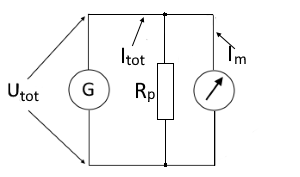# Parallel resistance of an ammeter

Calculators and formulas for calculating the parallel resistance of an ammeter

## Ammeter measuring range extension

This function calculates a parallel resistance to extend the measuring range of an ammeter. In principle, however, the function can also be used for any other component, whose current flow is to be reduced.

For the calculation, the ammeter current and the total current must be known. The voltage or resistance of the ammeter must also be specified. The input of the ammeter resistance is preset; it can be switched to voltage with the pull-down menu.

Parallel resistance calculator

 Input Total current mA A Ammeter current mA A Ammeter resistance Ammeter voltage mΩ Ω kΩ MΩ mV V Decimal places 0 1 2 3 4 6 8 10 Result Parallel resistor Power of Rp#### Calculation of the parallel resistance via the ammeter voltage

$$\displaystyle R_p=\frac{U_{tot}}{I_{tot}-I_m}$$

#### Calculation of the parallel resistance via the ammeter resistance

$$\displaystyle R_p=\frac{R_m}{n-1}$$       $$\displaystyle n=\frac{I_{tot}}{I_m}$$

#### Legend

 $$\displaystyle U_{tot}$$ Total voltage / ammeter voltage $$\displaystyle I_{tot}$$ Total current $$\displaystyle I_{m}$$ Current in the ammeter $$\displaystyle R_{m}$$ Ammeter resistance $$\displaystyle R_{p}$$ Value of the parallel resistance $$\displaystyle P_{p}$$ Power / load capacity of the parallel resistor